### Electricity - Test Papers

CBSE Test Paper-01

Chapter 12 Electricity and its Effects

1. The rest positions of the needles in a millimeter and voltmeter not in use are as shown in Fig a. when a student used these in his experiment, the readings of the needle are in the positions shown in Fig. B. The corrected values of current and voltage in the experiment are (1)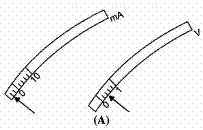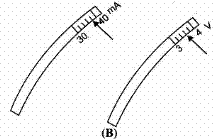1. 34 mA and 4.0 V
2. 42 mA and 4.0 V
3. 34 mA and 3.2 V
4. 42 mA and 3.2 V
2. A student uses as battery of adjustable voltage 0-6 V. she has to perform an experiment to determine the equivalent resistance of two resistors when connected in parallel using two resistors of value 3 ohm and 6 ohm. The best choice of combination of voltmeter and ammeter to be used in the experiment is (1)
1. Ammeter of range 0-5 A and voltmeter of range 0-2 V
2. Ammeter of range 0-2 A and voltmeter of range 0-10 V
3. Ammeter of range 0-5 A and voltmeter of range 0-5 V
4. Ammeter of range 0-5 A and voltmeter of range 0-10 V
3. A heater joined in parallel with a 60 W bulb is connected to the mains. If the 60 W bulb is replaced by a 100 W bulb, the rate of heat produced by the heater will be: (1)
1. More
2. Remains the same
3. None of these
4. Less
4. Students A and B connected the two resistors R1 and R2 given to them in the manners shown below :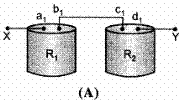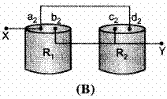and then insert at X and Y into the measuring circuit shown below :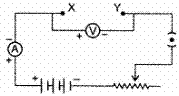We can say that (1)
1. both the students will determine the equivalent resistance of the series combination of R1 and R2.
2. Student A will determine the equivalent resistance of the series combination while student B will determine the equivalent resistance of the parallel combination of R1 and R2.
3. both the student will determine the equivalent resistance of the parallel combination of R1 and R2.
4. student A will determine the equivalent resistance of the parallel combination while student B will determine the equivalent resistance of the series combination of R1 and R2.
5. Out of the two circuits shown here, the two resistors R1 and R2 have been correctly connected in series in (1)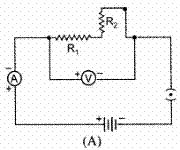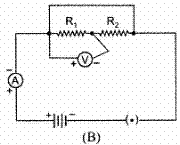1. circuit A only
2. circuit B only
3. neither of the two circuits
4. both circuits A and B
6. Define the unit of electric current. (1)
7. How does the mass of wire changes when the current is flowing in the wire ? (1)
8. How many joule are there in 1 kWh? (1)
9. How is a voltmeter connected in the circuit to measure potential difference between two points? (1)
10. Heat is generated continuously in an electric heater, but the temperature of its element becomes constant after some time. Why? (3)
11. The values of the current I flowing in a given resistor for corresponding values of potential difference V across the resistor are given below:
 I (amperes) 0.5 1 2 3 4 V (volts) 1.6 3.4 6.7 10.2 13.2
Plot a graph between V and I and calculate the resistance of that resistor. (3)
12. A heater coil is rated 100 W, 200 V. It is cut into two identical parts. Both parts are connected together in parallel to the same source of 200 V. Calculate the energy liberated per second in the new combination. (3)
13. A hot plate of an electric oven connected to a 220V line has two resistance coils A and B, each of 24 Ω resistance, which may be used separately, in series or in parallel. what are currents in three cases? (3)
14. (a) Define electrical energy with the S.I. unit?
(b) A household uses the following electric appliance:
(i) Refrigerator of rating 400Wfor ten hours each day.
(ii) Two electric fans of rating 80W each for twelve hours each day.
(iii) Six electric tubes of rating 18W each for six hours each day.
Calculate the electricity bill of the household for the month of June if the cost per unit of electric energy is $₹3.00$ (5)
15. A copper wire has diameter 0.5 mm and resistivity of $1.6×{10}^{-8}\mathrm{\Omega }m$. What will be the length of this wire to make its resistance 10 Q? How much does the resistance change if the diameter is doubled? (5)

CBSE Test Paper-01
Chapter 12 Electricity and its Effects

1. 42 mA and 3.2 V
Explanation: The miliammeter and the voltmeter has zero error and least count. Milliammeter has negative error. Voltmeter has positive error.
1. Ammeter of range 0-5 A and voltmeter of range 0-10 V
Explanation: Voltmeter has to read beyond 6 V and with 0-2A range, ammeter will have a better least count but net current drawn for 6V is 3A.
1. Remains the same
Explanation: Power (P) is the rate at which electric energy is lost (dissipated) or consumed in an electrical device due to resistance (R). $P\phantom{\rule{thinmathspace}{0ex}}=\frac{{V}^{2}}{R}$
The rate of heat produced by the heater will remain the same since 'V' remains the same, and the resistance 'R' of the heater remains the same.
1. Student A will determine the equivalent resistance of the series combination while student B will determine the equivalent resistance of the parallel combination of R1 and R2.
Explanation: c1, b1 is the common node in A so series b2, c2 pair and a2, d2 pair of points are at same potential. So parallel in B.
1. circuit A only
Explanation: In circuit B, the voltmeter is connected across R1 only. So the potential across the combination is not measured.
1. The SI unit of electric current is ampere (A). When 1 coulomb of electric charge flows through any cross-section of a conductor in 1 second, the electric current flowing through it is said to be 1 ampere.
1 ampere(A) = $\frac{1\mathrm{c}\mathrm{o}\mathrm{u}\mathrm{l}\mathrm{o}\mathrm{m}\mathrm{b}\left(\mathrm{C}\right)}{1\mathrm{s}\mathrm{e}\mathrm{c}\mathrm{o}\mathrm{n}\mathrm{d}\left(\mathrm{s}\right)}$

2. It remains the same.
3. 1kWh = 1,000 Ω × 1h = 1,000 J/S × 3600S
1kWh = 3,600,000 J = 3.6 ×106 J

4. Voltmeter is always connected in parallel to the ends of the resistor across which the potential difference is required to be measured.

5. When the temperature of the heater becomes greater than the temperature of the surroundings, some of the heat is lost to the surroundings in the form of thermal radiations and through convection. After some time,the rate at which heat is being produced becomes equal to the rate of which heat is lost. Hence, the temperature of the element becomes constant. This happens because an equilibrium or steady state is reached.

6. Graph between I and V is as shown in figure. Since graph is almost a straight line, the slope of line between any two points give the resistance. Slope of say OB is:
R = $\frac{AB}{OA\phantom{\rule{thickmathspace}{0ex}}}\phantom{\rule{thickmathspace}{0ex}}=\phantom{\rule{thickmathspace}{0ex}}\frac{BC-AC}{DA-OD}$
$\frac{AB}{OA\phantom{\rule{thickmathspace}{0ex}}}\phantom{\rule{thickmathspace}{0ex}}=\phantom{\rule{thickmathspace}{0ex}}\frac{12-1.6}{3.3-0.5}=\frac{10.4}{2.8}=\phantom{\rule{thickmathspace}{0ex}}\frac{104}{28}$
$R=\phantom{\rule{thickmathspace}{0ex}}3.7O\phantom{\rule{thickmathspace}{0ex}}$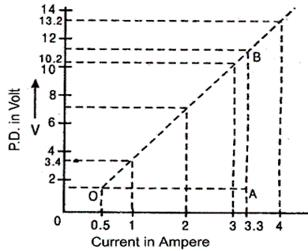7. Resistance of heater coil,
R = $\frac{{V}^{2}}{P}$
$\frac{{\left(200\right)}^{2}}{100}$
$\frac{200×200}{100}$
$400\mathrm{\Omega }$
As the coil is cut into two identical parts, resistance of each part= $\frac{400}{2}$ = $200\mathrm{\Omega }$
When connected in parallel, the net resistance is given by
$\frac{1}{{R}_{p}}$ = $\frac{1}{200}+\frac{1}{200}$
$⇒\frac{1}{{R}_{p}}=\frac{2}{200}$
$\therefore {R}_{p}$ = $100\mathrm{\Omega }$
Energy liberated = $P×t$
$\frac{{V}^{2}}{R}×t=\frac{{\left(200\right)}^{2}}{100}×1$
= 400J
$\therefore$ Energy liberated per second in the new combination is 400J

8. Each has a resistance 24 Ω when used separately.
Isp = $\frac{220}{24}$ =9.17A when used separately.
when used in series Rs = 24 + 24 = 48
Isr =$\frac{220}{48}$= 4.58A when used in series.
when used in parallel Rp = 12 Ω $\left[\frac{1}{{R}_{p}}=\phantom{\rule{thickmathspace}{0ex}}\frac{1}{24}+\frac{1}{24}\phantom{\rule{thickmathspace}{0ex}}0r\phantom{\rule{thickmathspace}{0ex}}{R}_{p}\phantom{\rule{thickmathspace}{0ex}}=12\mathrm{\Omega }\right]$
Ipr = $\frac{220}{12}$= 18.33A when used in prallel.

1. The work done by a source of electricity to maintain current in a circuit is known as electrical energy. Its S.I. unit is joule.
1. Electricity consumed by refrigerator in one day
= power $×$ time
= 400W $×$ 10h
= 4000Wh
= 4kWh
2. Electricity consumed by 2 electric fans in 1 day
= power $×$ time
= 2 $×$ 80W $×$ 12h
= 1920Wh
= 1.92kWh
3. Electricity consumed by 6 electric tubes in 1 day
= 6 $×$ 18W $×$ 6h
= 648wh = 0.648 kWh
Total energy consumed in one day
= 4+1.92+0.648 = 6.548 kWh
Total energy consumed in one month
= 6.568 $×$ 30
= 197.04 kWh
Cost of 1 unit (kWh) $=₹3.00$
$\therefore$Cost of 197.04 kWh = 197.04 $×$3
$\therefore$ The required electricity bill $=₹591.12$
9. (i) diameter, d=0.5 mm
Radius, r = $\frac{0.5}{2}mm$ = 0.25 mm
$\frac{0.25}{1000}m$
$0.25×{10}^{-3}m$
Area of cross-section, A = $\pi {r}^{2}$
$\frac{22}{7}×\left(0.25×{10}^{-3}{\right)}^{2}$
$0.1964×{10}^{-6}{m}^{2}$
Resistance, R=10$\mathrm{\Omega }$, Resistivity, $\rho$ = $1.6×{10}^{-8}\mathrm{\Omega }m$, Length, l=?
We know that, R = $\frac{\rho l}{A}$
l = $\frac{RA}{\rho }$
$\frac{10×0.1964×{10}^{-6}}{1.6×{10}^{-8}}$
$\frac{1964}{16}$ = 122.75 m
(ii) The area of cross-section,
${A}_{1}=\pi {r}^{2}$
$\pi {\left(\frac{d}{2}\right)}^{2}$ = $\frac{\pi {d}^{2}}{4}$
Its resistance, ${R}_{1}=\frac{\rho l}{{A}_{1}}$ = $\frac{4\rho l}{\pi {d}^{2}}$
$\because$Th e diameter is doubled, new area of cross-section,
${A}_{2}=\pi {\left(\frac{2d}{2}\right)}^{2}$ = $\pi {d}^{2}$
Its resistance, ${R}_{2}=\frac{\rho l}{{A}_{2}}$ = $\frac{\rho l}{\pi {d}^{2}}$
From (1) and (2), we have
$\frac{{R}_{2}}{{R}_{1}}=\frac{\rho l}{\pi {d}^{2}}×\frac{\pi {d}^{2}}{4pl}$ = $\frac{1}{4}$
or, ${R}_{2}=\frac{1}{4}{R}_{1}$
Thus, on doubling the diameter, the area of cross-section becomes 4 times and the resistance becomes one-fourth. Hence, the new resistance is